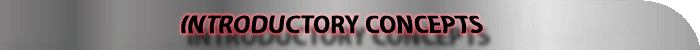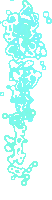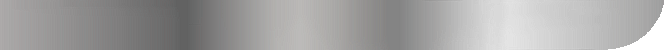TOWERS OF HANOI

 Towers of Hanoi is a recursive algorithm:   A method that is defined in terms of itself is called recursive. Recursion is a powerful design method that results in elegant and efficient algorithms. A recursive algorithm always consists of a base case and a recursive call usually with smaller or simpler arguments. It is very important to ensure that recursion terminates (i.e. base case is reached for any imput) Further examples of recursive algorithms include fibonacci, permutations as well as a number of other algorithms.   The Algorithm Pseudocode:   When current and final are the initial and final states, the algorithm is as follows:     solve ( current, final )     {      let max be the number of disks      let dest be the final place of max      let disk = max      repeat           while disk > 0 do              if disk is already on dest,              or, moving it succeeds then                   if disk = max then                        decrement max by 1                        if max = 0 then                          return    // done                        end if                       let dest be the final place of max                   end if                           else                   let dest be the alternative place between dest and                   the current place of disk              end if               decrement disk by 1           end while           let p and q be the places different of dest           let disk be the smaller of the disks on top of p and q          let dest be the place between p and q with greater disk on top          end repeat     }   Solving recurrences, in other words, obtaining asymptotic "omega" or "O" bounds on the solution can be attained using the substitution method. Here, a bound can be estimated and then a mathematical induction is used to prove the estimate correct. The iteration method converts the recurrence into a summation and then relies on techniques for bounding summations to solve the recurrence. The master method provides bounds for recurrences of the form T(n) = aT(n/b) + f(n), where a >= 1, b > 1, and f(n) is a given function. It requires memorization of three cases, but once that is done, determining asymptotic bounds for many simple recurrences is easy. The recursion tree A variation of the substitution method which implements visual representation (Please see text book).
 Algorithm In 1883, Edouard Lucas invented, or perhaps reinvented, one of the most popular puzzles of all times - the Tower of Hanoi. It is still used in many computer science textbooks to demonstrate  writing a recursive algorithm or program. The rules of the puzzle are as follows: There are three pegs: A, B and C. There are n disks. The number n is constant while working the puzzle. All disks are of different size. The disks are initially stacked on peg A so that they increase in size from the top to the bottom. The goal of the puzzle is to transfer the entire tower from  peg A to one of the other pegs. One disk at a time can be moved from the top of a stack either to an empty peg or to a peg with a disk larger than itself on the top of its stack.